# Boundary Conditions on the Fields at Interfaces - Electromagnetic Theory, CSIR-NET Physical Sciences Physics Notes | EduRev

## Physics : Boundary Conditions on the Fields at Interfaces - Electromagnetic Theory, CSIR-NET Physical Sciences Physics Notes | EduRev

The document Boundary Conditions on the Fields at Interfaces - Electromagnetic Theory, CSIR-NET Physical Sciences Physics Notes | EduRev is a part of the Physics Course Physics for IIT JAM, UGC - NET, CSIR NET.
All you need of Physics at this link: Physics

Boundary conditions for the normal components of the ﬁelds

When an electromagnetic ﬁeld faces an abrupt change in the permittivity and permeability, certain conditions on electric and magnetic ﬁelds on the interface are to be respected for the continuity. These conditions of continuity are known as the boundary conditions for the electromagnetic ﬁeld. Consider the pillbox in the following ﬁgure where two diﬀerent media are characterised by their permittivities and permeabilities, viz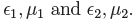The interface is shown with a curved surface. The height of the pillbox is h and the two ﬂat surfaces of the pillbox in two diﬀerent media are shown by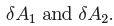We start with the Maxwell’s equation,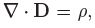and integrate it over the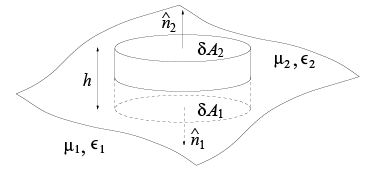Fig. 11.1: Pillbox on the interface of two media

pillbox.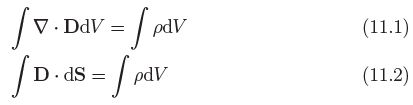where S is the total surface area of the pillbox. Now the left hand side of the above equation is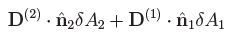+ ﬂux through the curved surface of the pillbox, (11.3)
where the superscripts identify the ﬁelds in diﬀerent media We now reduce the height of the pillbox eventually making it to zero. In this case the area of the curved surface reduces to zero and hence the ﬂux through it is also zero.
Since there is a ﬁnite charge inside the box,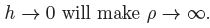So in this limit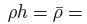finite and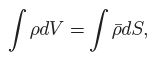where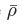is surface charge density on the interface.
In this situation,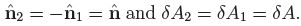The right hand side of the equation for small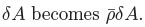Using the above relations in (11.2), we have the condition,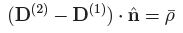(11.4)

The above condition says that there is an abrupt jump in the normal component of the displacement vector while crossing the medium if there is a non zero surface charge density on the interface. Similarly we proceed with the Maxwell equation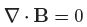and obtain the following boundary condition,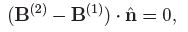(11.5)

which says the normal component of the magnetic ﬁled is always continuous.

Boundary conditions for the tangential components of the ﬁelds

Let us consider the following small closed curve, P QRS , across the interface of two media. The area, PQRS, has the unit vector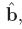which is normal to the surface P QRS .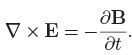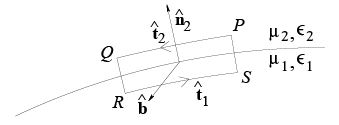Fig. 11.2: Closed curve across the interface of two media

The scalar product of the above equation withis integrated over the surface PQRS .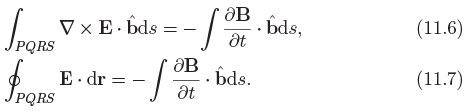The left hand side of equation (11.9) is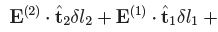contributions from QR and S P ,

where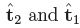are the unit vectors along P Q and RS respectively.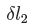and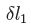are the lengths of P Q and RS respectively.

Now in the limit QR → 0 and S P → 0 the right hand side of the equation (11.9) vanishes(as the area of P QRS vanishes) as well as in the left hand side the contributions from QR and S P also vanish and then we have (with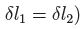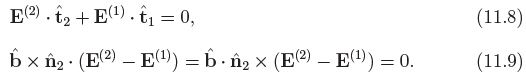Since the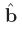arbitrary we get the following condition for the tangential component of the electric ﬁeld (for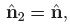, normal to the surface)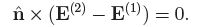(11.10)

Similarly proceeding with the Maxwell’s equation (3.4), we get the condition on the tangential component of the H ﬁeld as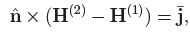(11.11)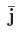is the surface current density.

Offer running on EduRev: Apply code STAYHOME200 to get INR 200 off on our premium plan EduRev Infinity!

159 docs

,

,

,

,

,

,

,

,

,

,

,

,

,

,

,

,

,

,

,

,

,

,

,

,

;